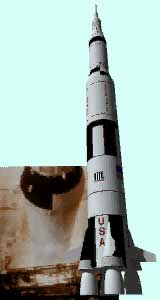Ch 4. Particle Momentum and Impulse Multimedia Engineering Dynamics Impulse & Momentum Consv. Linear Momentum Impact AngularMomentum MassFlow
 Chapter - Particle - 1. General Motion 2. Force & Accel. 3. Energy 4. Momentum - Rigid Body - 5. General Motion 6. Force & Accel. 7. Energy 8. Momentum 9. 3-D Motion 10. Vibrations Appendix Basic Math Units Basic Equations Sections Search eBooks Dynamics Fluids Math Mechanics Statics Thermodynamics Author(s): Kurt Gramoll ©Kurt GramollDYNAMICS - CASE STUDY IntroductionProblem Graphic When designing rockets to transport a given payload into space, rocket scientists must determine the optimal mass of fuel for the rocket to carry. How can they determine the maximum velocity a rocket will attain based on the amount of fuel it carries? What is known: The total initial mass of a rocket and its fuel is mo. The fuel has an initial mass mf, and burns at a constant rate of dmf/dt = c. The fuel is expelled from the rocket at a constant speed vf relative to the rocket. The rocket is fired vertically from rest. Drag resistance of the air may be neglected. Question What is the velocity of the rocket in terms of given parameters once it has burned all of its fuel? Approach Use conservation of linear momentum to determine the force exerted on an object as a result of emitting a continuous flow of mass. Use Newton's Second Law to derive an equation relating the sum of forces acting on the rocket to the rocket's acceleration. Integrate to determine the rocket's velocity.

Practice Homework and Test problems now available in the 'Eng Dynamics' mobile app
Includes over 400 problems with complete detailed solutions.
Available now at the Google Play Store and Apple App Store.